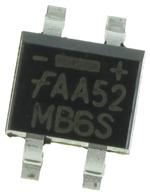< id="6e2kg">< id="6e2kg">< id="6e2kg">< id="6e2kg">< id="6e2kg">< id="6e2kg">< id="6e2kg">< id="6e2kg">< id="6e2kg">< id="6e2kg">< id="6e2kg">< id="6e2kg">< id="6e2kg">< id="6e2kg">< id="6e2kg">< id="6e2kg">< id="6e2kg">< id="6e2kg">< id="6e2kg">< id="6e2kg">< id="6e2kg">< id="6e2kg">< id="6e2kg">< id="6e2kg">< id="6e2kg">< id="6e2kg">< id="6e2kg">< id="6e2kg">< id="6e2kg">< id="6e2kg">< id="6e2kg">< id="6e2kg">< id="6e2kg">< id="6e2kg">< id="6e2kg">< id="6e2kg">< id="6e2kg">< id="6e2kg">< id="6e2kg">< id="6e2kg">< id="6e2kg">< id="6e2kg">< id="6e2kg">< id="6e2kg">< id="6e2kg">< id="6e2kg">< id="6e2kg">< id="6e2kg">< id="6e2kg">< id="6e2kg"> < id="6e2kg">< id="6e2kg">< id="6e2kg">< id="6e2kg">< id="6e2kg">< id="6e2kg">< id="6e2kg">< id="6e2kg">< id="6e2kg">< id="6e2kg">< id="6e2kg">< id="6e2kg">< id="6e2kg">< id="6e2kg">< id="6e2kg">< id="6e2kg">< id="6e2kg">< id="6e2kg">< id="6e2kg">< id="6e2kg">< id="6e2kg">< id="6e2kg">< id="6e2kg">< id="6e2kg">< id="6e2kg">< id="6e2kg">< id="6e2kg">< id="6e2kg">< id="6e2kg">< id="6e2kg">< id="6e2kg">< id="6e2kg">< id="6e2kg">< id="6e2kg">< id="6e2kg">< id="6e2kg">< id="6e2kg">< id="6e2kg">< id="6e2kg">< id="6e2kg">< id="6e2kg">< id="6e2kg">< id="6e2kg">< id="6e2kg">< id="6e2kg">< id="6e2kg">< id="6e2kg">< id="6e2kg">< id="6e2kg">< id="6e2kg"> < id="6e2kg">< id="6e2kg">< id="6e2kg">< id="6e2kg">< id="6e2kg">< id="6e2kg">< id="6e2kg">< id="6e2kg">< id="6e2kg">< id="6e2kg">< id="6e2kg">< id="6e2kg">< id="6e2kg">< id="6e2kg">< id="6e2kg">< id="6e2kg">< id="6e2kg">< id="6e2kg">< id="6e2kg">< id="6e2kg">< id="6e2kg">< id="6e2kg">< id="6e2kg">< id="6e2kg">< id="6e2kg">< id="6e2kg">< id="6e2kg">< id="6e2kg">< id="6e2kg">< id="6e2kg">< id="6e2kg">< id="6e2kg">< id="6e2kg">< id="6e2kg">< id="6e2kg">< id="6e2kg">< id="6e2kg">< id="6e2kg">< id="6e2kg">< id="6e2kg">< id="6e2kg">< id="6e2kg">< id="6e2kg">< id="6e2kg">< id="6e2kg">< id="6e2kg">< id="6e2kg">< id="6e2kg">< id="6e2kg">< id="6e2kg">型号： MB6S 厂商：Fairchild Semiconductor 标准：分类： 半导体 ， 分离式半导体 描述： bridge rectifiers 0.5A bridge PDF： 预览 报错 收藏 赞

Datasheet下载地址

 本地下载 >> 厂商下载2 >> 第三方平台下载 >>

MB6S的详细信息

Manufacturer： Fairchild Semiconductor Bridge Rectifiers Yes Single Phase Bridge 600 V 420 V 0.5 A 35 A 1 V 5 uA 1.4 W + 150 C 4.9 mm 4.2 mm 2.7 mm SMD/SMT SOIC-4 Reel Fairchild Semiconductor - 55 C MB6S 3000 MB6S_NL 228 mg
April 2014
MB1S - MB8S
0.5 A Bridge Rectifiers
Description
Features
The MB family of bridge rectifiers is a 0.5 A rectifier fam-
ily that achieves high surge current absorption within a
very small foot print. Within its small 35 mm form factor,
? Low-Leakage
? Surge Overload Rating: 35 A peak
? Ideal for Printed Circuit Board
? UL Certified: UL #E258596
2
the MB family shines in its surge capability. In order to
absorb high surge currents, the design supports a 35 A
2
2
I
rating and a 5.0 A Sec I T rating. Devices in the
FSM
family are also rated to breakdown voltages of up to
1000 V. These features make the MB family ideal for
small power supplies that need a little extra surge capa-
bility.
4
3
For higher I
current ratings, lower profile packaging,
FAV
or lower V values, explore the Fairchild MDB family of
F
bridge rectifiers. For improved V and efficiency values
in the MB package or even higher surge capability, ask
~
~
F
-
+
2
1
SOIC-4
Polarity symbols molded
or mark on body
Ordering Informations
Part Number
MB1S
Marking
MB1S
MB2S
MB4S
MB6S
MB8S
Package
Packing Method
MB2S
MB4S
SOIC-4
Tape and Reel
MB6S
MB8S
? 2007 Fairchild Semiconductor Corporation
MB1S - MB8S Rev. 1.2.3
www.fairchildsemi.com
1